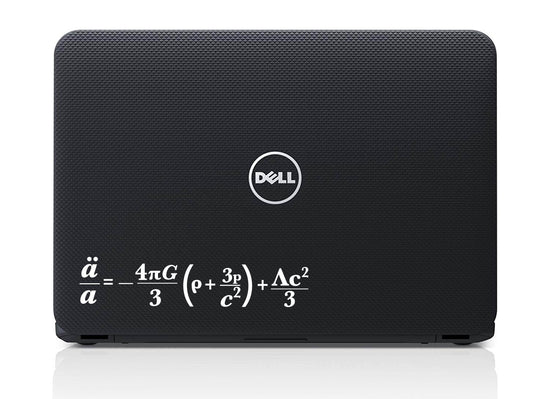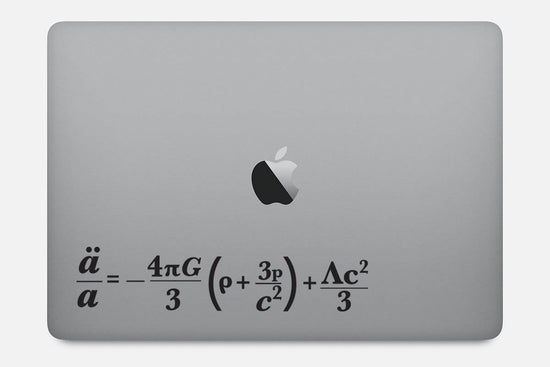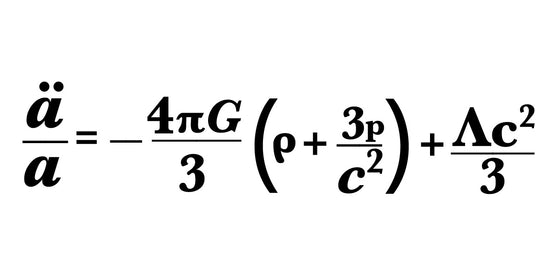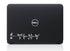Buttered Kat

# Friedmann Equation Decal Sticker

The Friedmann equation describes the expansion of space in homogeneous and isotropic models of the universe within the context of general relativity.
Where the ratio a:/a= H ( the Hubble constant),G, Λ, and c are universal constants (G is Newton's gravitational constant, Λ is the cosmological constant, and c is the speed of light in vacuum). k is constant throughout a particular solution, but may vary from one solution to another. a, H, ρ, and p are functions of time. ρ, and p are the density and pressure, respectively.

It can be applied to any smooth surface, comes in two sizes and seven colors. Contact us if you require custom size or color.

• chemistry
• Chemistry decal
• computer decal
• computer sticker
• Decal
• equation
• Physics
• Physics Decal
• science
Regular price
\$11.00
Sale price
\$11.00
Regular price

••••••••••••••••